# Electronics and Communication Engineering - Exam Questions Papers

46.

The transfer function of a system is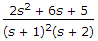. The characteristic equation of the system is :

 A. 2s2 + 6s + 5 = 0 B. (s + l)2 (s + 2) = 0 C. 2s2 + 6s + 5 + (s + 1 )2(s + 2) = 0 D. 2s2 + 6s + 5 - (s + l)2(s + 2) = 0

Explanation:

Characteristic equation = 1 + GH = 0

(s + 1)2(s + 2) + (2s2 + 6s + s) = 0

Apply R-H.

47.

Given f = GEF + KHIJ + LMON + TUVWXYZ and

1. E + F + G = LMN
2. V + W = U . Z . Y . X . T
3.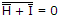NAND B
4. (UVW ⊕ WVU) = KJ(XY ⊕ XY)

Then f is equivalent to

 A. 0 B. 1 C. EF + UZ + HI D. None of these

Explanation:

(UVW ⊕ WVU) = 1 = KJ(XY ⊕ XY)

KJ = 1NAND B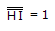HI = 1

Since f = GEF + 1 + LMON + TUVWXYZ = 1.

48.

If V = 4 in the figure, the value of IS is given by,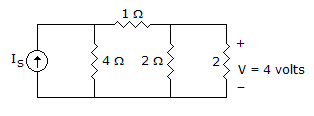A. 6 A B. 5/2 A C. 12 A D. None of these

Explanation:

Using Nodal analysis

At node V1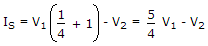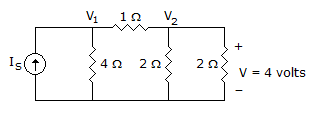At node V2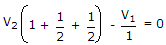2V2 - V1 = 0

V1 = 2V2 Put V2 = V = 4

V1 = 8 V

Put in equation (i), IS =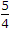x 8 - 4 = 10 - 4 = 6 A.

49.

Choose correct option for a stable system :

1. Roots of the characteristic equation of the system are real and negative
2. Area within the impulse response is finite

 A. I is true, II is true B. I is true, II is false C. I is false, II is true D. I is false, II is false

Explanation:

From Statement 1: Roots of the characteristics equation of the system are real and negative i.e. the poles are on the left half plane.

Hence the system is stable.

From Statement 2: Area within the impulse response is finite i.e. it is finite duration signal. It produces a bounded output. Hence system is stable.

So both the statements are true.

50.

The number of distinct Boolean expressions of 3 variables is

 A. 8 B. 256 C. 64 D. 7